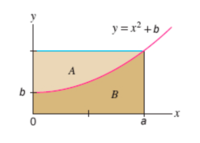# Volume of revolution

#### rir0302

##### New memberFind the volume of the solid obtained by rotating the region A in the figure about x=−6.
Assume b=6, a=2.

Then, find the volume of the solid obtained by rotating the region 𝐵 in the figure about 𝑥=−3.
Assume b=4 and a=2.

I think I need help with the basic concept too because I'm stuck. For the first part, I tried doing the doing the disk formula for the equation, but I don't understand how to rotate around x=-6. Could someone show me the steps for the question? Thanks.

#### MarkFL

##### Super Moderator
Staff member
Hello, and welcome to FMH!Let's let the axis of rotation be:

$$\displaystyle x=-k$$ where $$0<k$$

Using the shell method, an element of the volume of the solid can be given as:

$$\displaystyle dv=2\pi(k+x)y\,dx=2\pi(x^3+kx^2+bx+bk)\,dx$$

Hence:

$$\displaystyle V=2\pi\int_0^a x^3+kx^2+bx+bk\,dx=2\pi\left[\frac{1}{4}x^4+\frac{k}{3}x^3+\frac{b}{2}x^2+bkx\right]_0^a=\frac{\pi a}{6}\left(3a^3+4a^2k+6ab+12bk\right)$$

Now we have a formula into which we can place the parameters $$a,\,b,\,k$$.

See if you can derive a similar formula for region A.

Last edited: